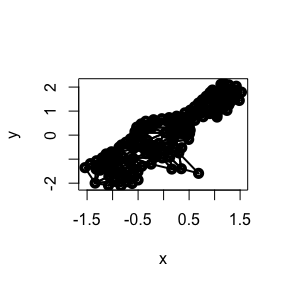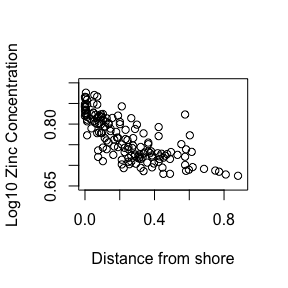# Meuse

library(SpatialfdaR)
#>
#> Attaching package: 'fda'
#> The following object is masked from 'package:graphics':
#>
#>     matplot
#> Loading required package: geometry

The Meuse data analyzed in this vignette are locations along the bank of the river Meuse in Belgium where the amount of zinc in the soil was recorded. The river loops sharply in this location, and the soil pollution by heavy metals used in nearby industry was of concern.

The list object MeuseData.rda contains the locations of soil samples, and these are also used as nodes in the mesh that will define the estimated surface displaying soil pollution. The mesh was built by using Delaunay mesh generation software, such as R functions geometry::delaunayn or RTriangle::triangulate. It should be appreciated that Delaunay meshes are often not unique.

The Delaunay mesh generated a number of triangles that were outside of the desired boundary because of the non-convexity of this boundary. These triangles were removed by hand to define the final mesh. The 155 points, the 52 edges and the 257 triangles are retrieved as follows:

p <- MeuseData$pts e <- MeuseData$edg
t <- MeuseData$tri np <- dim(p)[] ne <- dim(e)[] nt <- dim(t)[] covdata <- MeuseData$covdata

The edge data are not needed in this analysis.

The mesh is displayed by

plotFEM.mesh(p, t, nonum=TRUE)The river flows above the mesh from the upper right to the lower left, then around the sharp curve, and out below abnd beyond the projecting portion of the mesh.

The data used to define the surface is the log of the zinc level, and preliminary plots indicated that this variable was strongly correlated with the shorted distance from the river bank. The first variable is treated in the analysis as what is to be fit by the surface, and distance was treated as a covariate that provides further information about the estimated height of the log-zinc surface. These data are in the two-column matrix MeuseData$covdata, with distance in the first column and zinc level in the second. data <- matrix(0,nrow=np,ncol=2) data[,1] <- covdata[,1] data[,2] <- log(covdata[,2]) Dist <- as.matrix(covdata[,1]) Zinc <- as.matrix(log(covdata[,2])) LogZinc <- log10(Zinc) We can conduct a preliminary assessment of the log-zinc/distance covariation using lsList <- lsfit(Dist, LogZinc) print(paste("Regression coefficient =",round(lsList$coef,2)))
#>  "Regression coefficient = 0.81"
print(paste("Intercept =",round(lsList$coef,2))) #>  "Intercept = -0.2" and displayed by plot(Dist, LogZinc, type="p", xlim=c(0,0.9), ylim=c(0.65, 0.90), xlab = "Distance from shore", ylab = "Log10 Zinc Concentration") abline(lsList$int, lsList$coef)The first step in our surface estimation process is to define an FEM basis by using the “pet” architecture of the mesh as arguments: MeuseBasis <- create.FEM.basis(p, e, t) A hazard when the observations are all at the nodes is that the linear equation that must be solved in smoothing function cannot be solved because the left side is not of full rank. A simple way around that is to impose some penalty on the roughness of the solution by setting roughness penalty lambda to a positive value. Here is the command that produces a nice smooth surface by using lambda = 1e-4: smoothList <- smooth.FEM.basis(p, LogZinc, MeuseBasis, lambda=1e-4, covariates=Dist) LogZincfd <- smoothList$fd
df        <- smoothList$df gcv <- smoothList$gcv
beta      <- as.numeric(smoothList$beta) SSE <- smoothList$SSE

The following commands plot the fitted surface by using the powerful graphics capabilities of the rgl package.

Xgrid <- seq(-2.2, 2.2, len=21)
Ygrid <- seq(-2.2, 2.2, len=21)
plotFEM.fd(LogZincfd, Xgrid, Ygrid,
xlab="Latitude", ylab="Longitude", zlab="log10 Zinc Concentration")
par(op)

The coefficient for the covariate Dist is 1.83 and error sum of squares is 49.9. We can rerun the analysis without the contribution from Dist:

smoothList0 <- smooth.FEM.basis(p, LogZinc, MeuseBasis, lambda=1e-4)
SSE0        <- smoothList0\$SSE

Now we get the error sum of squares 59.8. The squared multiple correlation coefficient is R^2 = (59.8 - 49.9)/58.8 = 0.165, which indicates a relatively uninteresting contribution of the Dist variable to the fit to the data.

There are many ways to improve this analysis. The mesh has a lot of small triangles due to a large number of sampling positions in the lower left corner of the mesh. We can leave the nodes in as locations of the data, and replace p in the argument by loc which is identical to p. Then we might then remove a number of nodes in this densely sampled area so that the triangles that are defined have roughly the same areas as those elsewhere in the mesh. The number of rows i p will decrease, and we will rebuild the FEM basis using the create.FEM.basis function. This will define a smooth surface using smaller values of lambda.

In any case, the triangles needn’t be as small they are in this analysis for the purposes of examining the variation in the zinc deposits. Reducing the mesh density is a good way to go.